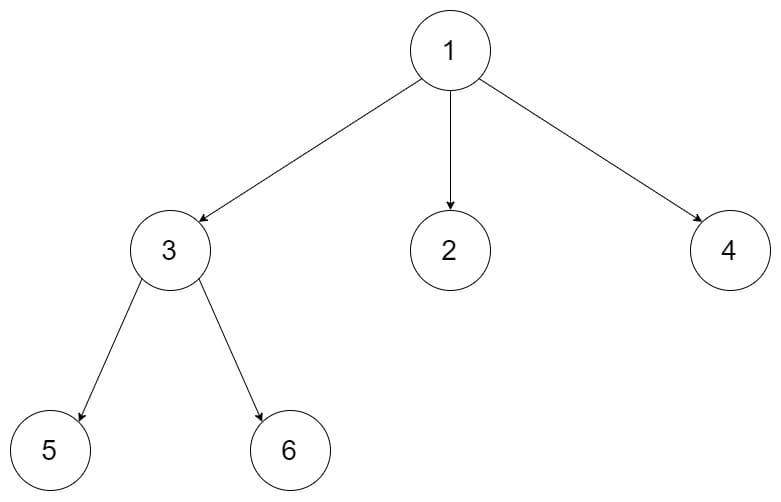# 429. N-ary Tree Level Order Traversal

Reference: LeetCode
Difficulty: Easy

## Problem

Given an n-ary tree, return the level order traversal of its nodes’ values. (ie, from left to right, level by level).

Example:Note:

• The depth of the tree is at most 1000.
• The total number of nodes is at most 5000.

## Analysis

### Recursion

The recursion is actually based on the preorder traversal.

Time: $O(N)$
Space: $O(N)$ to store all nodes.

### Iteration

In the foreach statement, if p.children is null, it would crash. However, if a node is in p.children, it can’t be null.

Time: $O(N)$
Space: $O(N)$ to store all nodes.

CommentJunhao Wang
a software engineering cat